# Topological Sort Using DFS

Problem Statement: Given a DAG( Directed Acyclic Graph ), print all the vertex of the graph in a topologically sorted order. If there are multiple solutions, print any.

Pre-req: DFS traversal, Graphs, Stack data structure.

Examples:

```Example 1:
Input: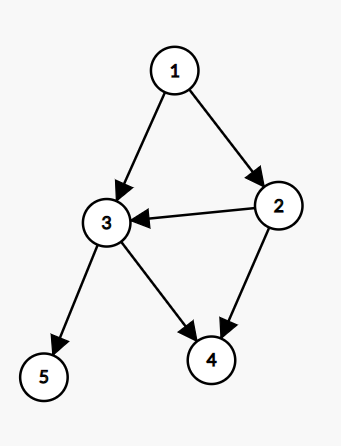Output:
One of the solutions is 1,2,3,5,4```
```Example 2:
Input: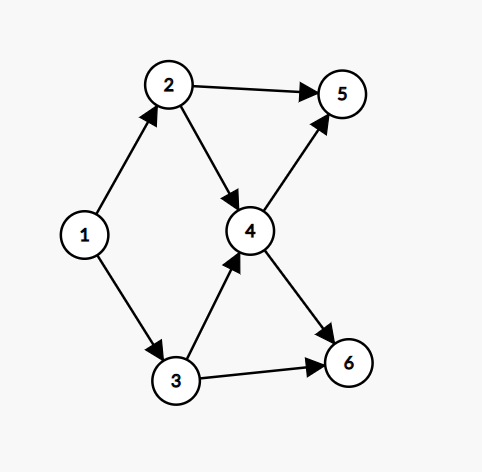Output: One of the solution is 1,2,3,4,5,6```

### Solution

Disclaimer: Don’t jump directly to the solution, try it out yourself first.

Intuition:

-> First of all let’s understand Topological Sorting. It means linear ordering of vertices such that there is an edge u—-> v, u appears before v in the ordering.

Suppose for a given graph,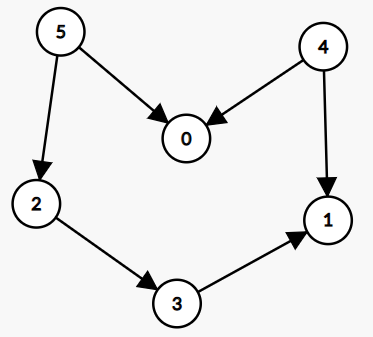Some of the possible Topological orders can be:

1.  5,4,2,3,1,0
2.  4,5,2,3,1,0

-> In both cases we can see, that

4->0 ( 4 appears before 0 ) , 5>3 ( 5 appears before 3 ), …

-> Similarly there can be multiple toposorts order for the given graph but the condition should be if there is an edge u->v then u should always appear before v.

-> Topological Sorting is applicable only for DAG(Directed Acyclic Graph). Why is it so?

Because of the following reasons:

1. For Undirected graphs ,only u->v is not applicable . It cannot be sure whether the edge is between u to v or v to u ( u-v ) .
2. In a cyclic graph there will always be a dependency factor . You cannot make sure that you can have linear ordering of vertices.

-> Finally, now you have a clear understanding of what Topological Sorting is. We will be using the DFS(Depth First Search) method to solve the problem. What we will be doing is for each vertex we will explore its adjacent vertex. After exploring, we will store the current vertex in a data structure to maintain Topo Sort.

Approach:

We will be using the following data structure to get Topo sort:

1. Visited Vector – To store visit of each vertex
2. Stack – To maintain the topo sort order.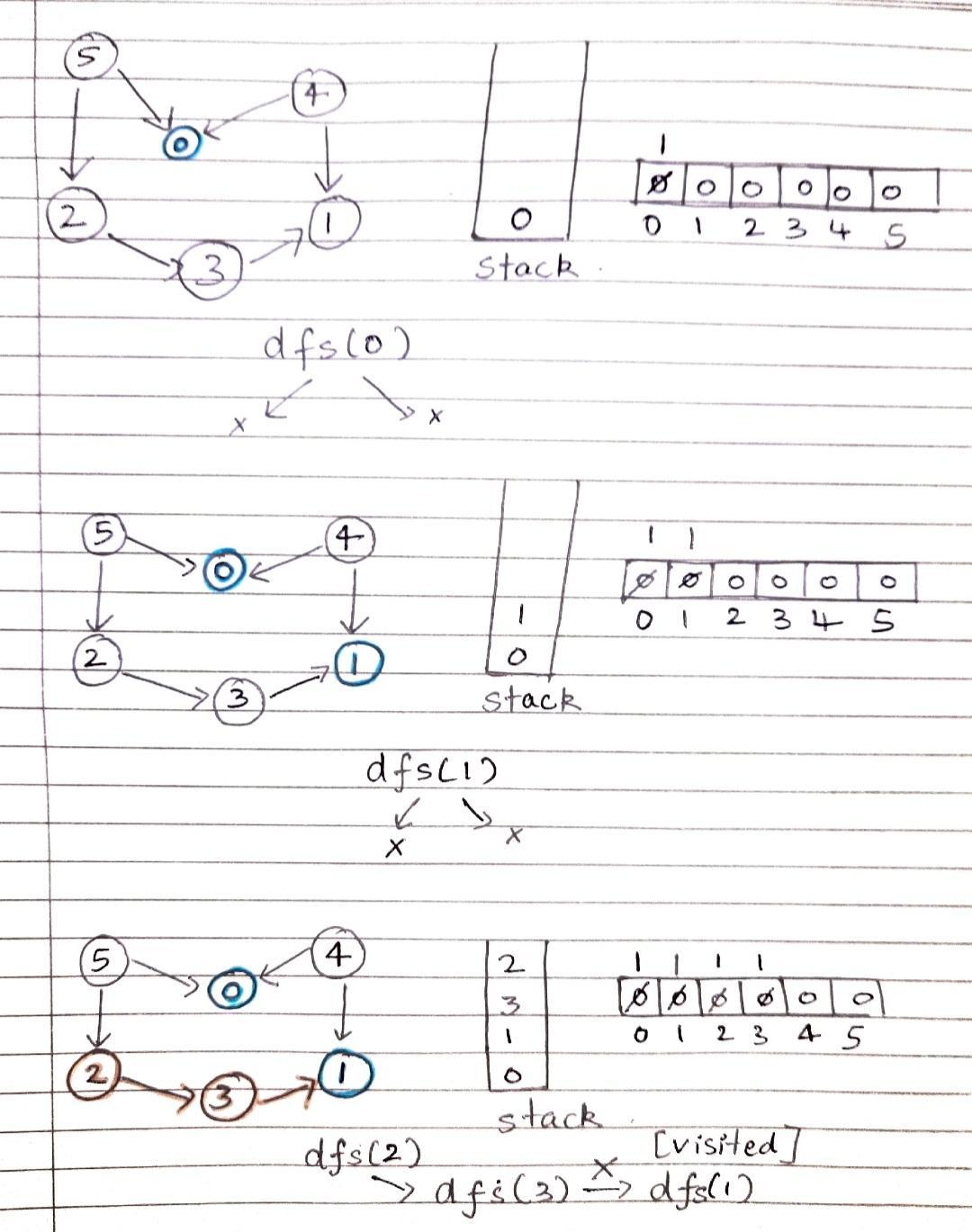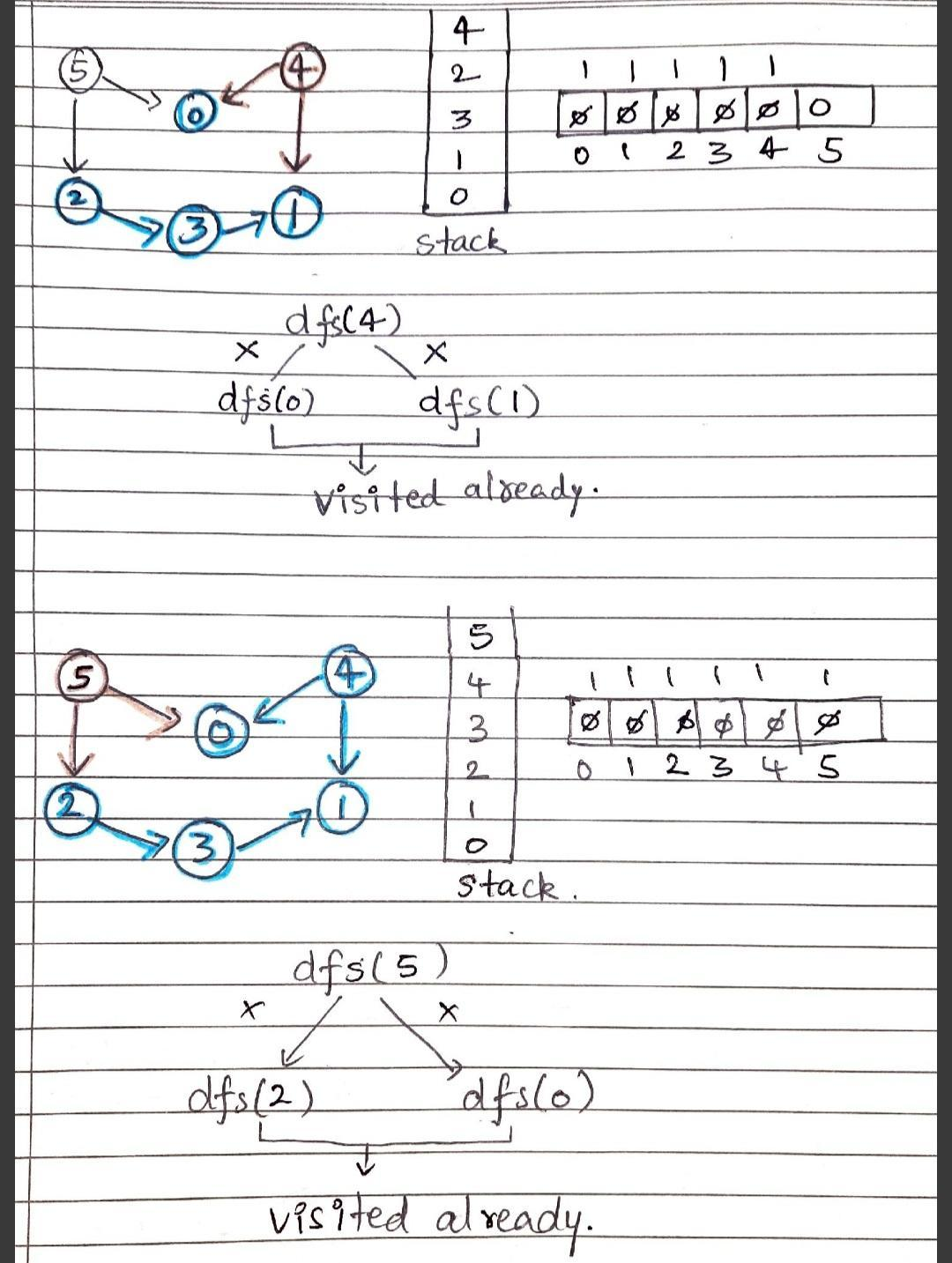Did you notice something while using Stack? Just because there was an edge from u to v. Dfs call will go from u to v. The 1st dfs (v) will get over first and then dfs(u). Here we are making sure that if u->v, then we will first push v into the stack and then u will be pushed. This is how Topological order is maintained in the Stack.

Code:

## C++ Code

``````#include <bits/stdc++.h>

using namespace std;

class Solution {
void findTopoSort(int node, vector < int > & vis, stack < int > & st, vector < int > adj[]) {
vis[node] = 1;

for (auto it: adj[node]) {
if (!vis[it]) {
findTopoSort(it, vis, st, adj);
}
}
st.push(node);
}
public:
vector < int > topoSort(int N, vector < int > adj[]) {
stack < int > st;
vector < int > vis(N, 0);
for (int i = 0; i < N; i++) {
if (vis[i] == 0) {
findTopoSort(i, vis, st, adj);
}
}
vector < int > topo;
while (!st.empty()) {
topo.push_back(st.top());
st.pop();
}

}
};

// { Driver Code Starts.
int main() {

int N = 6;

vector < int > adj[5 + 1];

Solution obj;
vector < int > res = obj.topoSort(6, adj);

cout << "Toposort of the given graph is:" << endl;
for (int i = 0; i < res.size(); i++) {
cout << res[i] << " ";
}

return 0;
}
``````

Output:

Toposort of the given graph is:
5 4 2 3 1 0

Time Complexity: O(N+E)

N = Number of node , E = Number of Edges

Space Complexity: O(N) + O(N)

Visited Array and Stack data structure. Both will be using O(N).

Auxiliary Space Complexity:  O(N)

Recursion call of DFS

## Java Code

``````import java.util.*;
class Solution {
static void findTopoSort(int node, int vis[], ArrayList<ArrayList<Integer>> adj, Stack<Integer> st) {
vis[node] = 1;
for(Integer it: adj.get(node)) {
if(vis[it] == 0) {
findTopoSort(it, vis, adj, st);
}
}
st.push(node);
}
static int[] topoSort(int N, ArrayList<ArrayList<Integer>> adj) {
Stack<Integer> st = new Stack<Integer>();
int vis[] = new int[N];

for(int i = 0;i<N;i++) {
if(vis[i] == 0) {
findTopoSort(i, vis, adj, st);
}
}

int topo[] = new int[N];
int ind = 0;
while(!st.isEmpty()) {
topo[ind++] = st.pop();
}
// for(int i = 0;i<N;i++) System.out.println(topo[i] + " ");
}
public static void main(String args[])
{
int n=6;
for(int i=0;i<n;i++)
{
ArrayList<Integer> arr=new ArrayList<>();
}

int res[] = topoSort(6, adj);

System.out.println("Toposort of the given graph is:" );
for (int i = 0; i < res.length; i++) {
System.out.print(res[i]+" ");
}
}
}``````

Output:

Toposort of the given graph is:
5 4 2 3 1 0

Time Complexity: O(N+E)

N = Number of node , E = Number of Edges

Space Complexity: O(N) + O(N)

Visited Array and Stack data structure. Both will be using O(N).

Auxiliary Space Complexity:  O(N)

Recursion call of DFS

Special thanks to Shreyas Vishwakarma for contributing to this article on takeUforward. If you also wish to share your knowledge with the takeUforward fam, please check out this article